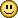cancel
Showing results for
Did you mean:

## very slow browsing

Community Veteran
Posts: 1,817
Thanks: 11
Registered: ‎30-07-2007

## very slow browsing

my net speed for some sites seams completely constipated.
I tried pinging the affected sites which are showing ttl in the region of 250ms including pn. google remains at only 55ms and remains useable.

Pinging plus.net [212.159.8.2] with 32 bytes of data:
Reply from 212.159.8.2: bytes=32 time=49ms TTL=250
Reply from 212.159.8.2: bytes=32 time=52ms TTL=250
Reply from 212.159.8.2: bytes=32 time=47ms TTL=250
Reply from 212.159.8.2: bytes=32 time=46ms TTL=250

also some timeouts

Pinging 212.159.9.2 with 32 bytes of data:  (PN)
Request timed out.
Reply from 212.159.9.2: bytes=32 time=43ms TTL=250
Reply from 212.159.9.2: bytes=32 time=42ms TTL=250
Reply from 212.159.9.2: bytes=32 time=41ms TTL=250
Ping statistics for 212.159.9.2:
Packets: Sent = 4, Received = 3, Lost = 1 (25% loss),
Approximate round trip times in milli-seconds:
Minimum = 41ms, Maximum = 43ms, Average = 42ms
Pinging bbc.co.uk [212.58.224.138] with 32 bytes of data:
Request timed out.
Reply from 212.58.224.138: bytes=32 time=36ms TTL=120
Reply from 212.58.224.138: bytes=32 time=36ms TTL=120
Reply from 212.58.224.138: bytes=32 time=35ms TTL=120
Ping statistics for 212.58.224.138:
Packets: Sent = 4, Received = 3, Lost = 1 (25% loss),
Approximate round trip times in milli-seconds:
Minimum = 35ms, Maximum = 36ms, Average = 35ms

I have rebooted but get the same slow speeds to these sites.

Ping statistics for 212.159.8.2:
Packets: Sent = 4, Received = 4, Lost = 0 (0% loss),
Approximate round trip times in milli-seconds:
Minimum = 46ms, Maximum = 52ms, Average = 48ms
Pinging google.co.uk [209.85.227.147] with 32 bytes of data:
Reply from 209.85.227.147: bytes=32 time=43ms TTL=55
Reply from 209.85.227.147: bytes=32 time=42ms TTL=55
Reply from 209.85.227.147: bytes=32 time=41ms TTL=55
Reply from 209.85.227.147: bytes=32 time=41ms TTL=55
Ping statistics for 209.85.227.147:
Packets: Sent = 4, Received = 4, Lost = 0 (0% loss),
Approximate round trip times in milli-seconds:
Minimum = 41ms, Maximum = 43ms, Average = 41ms
Jojo